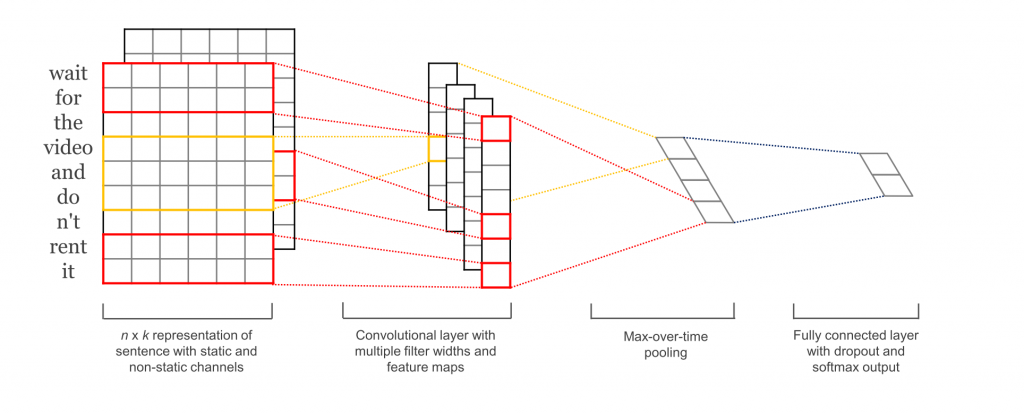Magnetic Fridge Poetry (source: Steve Johnson on Flickr)

When approaching problems with sequential data, such as natural language tasks, recurrent neural networks (RNNs) typically top the choices. While the temporal nature of RNNs are a natural fit for these problems with text data, convolutional neural networks (CNNs), which are tremendously successful when applied to vision tasks, have also demonstrated efficacy in this space.

In our LSTM tutorial, we took an in-depth look at how long short-term memory (LSTM) networks work and used TensorFlow to build a multi-layered LSTM network to model stock market sentiment from social media content. In this post, we will briefly discuss how CNNs are applied to text data while providing some sample TensorFlow code to build a CNN that can perform binary classification tasks similar to our stock market sentiment model.Figure 1. Sample CNN model architecture for text classification. Image by Garrett Hoffman, based on a figure from “Convolutional Neural Networks for Sentence Classification.”

We see a sample CNN architecture for text classification in Figure 1. First, we start with our input sentence (of length seq_len), represented as a matrix in which the rows are our words vectors and the columns are the dimensions of the distributed word embedding. In computer vision problems, we typically see three input channels for RGB; however, for text we have only a single input channel. When we implement our model in TensorFlow, we first define placeholders for our inputs and then build the embedding matrix and embedding lookup.

```# Define Inputs
inputs_ = tf.placeholder(tf.int32, [None, seq_len], name='inputs')
labels_ = tf.placeholder(tf.float32, [None, 1], name='labels—)
training_ = tf.placeholder(tf.bool, name='training')

# Define Embeddings
embedding = tf.Variable(tf.random_uniform((vocab_size, embed_size), -1, 1))
embed = tf.nn.embedding_lookup(embedding, inputs_)
```

Notice how the CNN processes the input as a complete sentence, rather than word by word as we did with the LSTM. For our CNN, we pass a tensor with all word indices in our sentence to our embedding lookup and get back the matrix for our sentence that will be used as the input to our network.

Now that we have our embedded representation of our input sentence, we build our convolutional layers. In our CNN, we will use one-dimensional convolutions, as opposed to the two-dimensional convolutions typically used on vision tasks. Instead of defining a height and a width for our filters, we will only define a height, and the width will always be the embedding dimension. This makes sense intuitively, when compared to how images are represented in CNNs. When we deal with images, each pixel is a unit for analysis, and these pixels exist in both dimensions of our input image. For our sentence, each word is a unit for analysis and is represented by the dimension of our embeddings (the width of our input matrix), so words exist only in the single dimension of our rows.

We can include as many one-dimensional kernels as we like with different sizes. Figure 1 shows a kernel size of two (red box over input) and a kernel size of three (yellow box over input). We also define a uniform number of filters (in the same fashion as we would for a two-dimensional convolutional layer) for each of our layers, which will be the output dimension of our convolution. We apply a relu activation and add a max-over-time pooling to our output that takes the maximum output for each filter of each convolution—resulting in the extraction of a single model feature from each filter.

```# Define Convolutional Layers with Max Pooling
convs = []
for filter_size in filter_sizes:
conv = tf.layers.conv1d(inputs=embed, filters=128, kernel_size=filter_size, activation=tf.nn.relu)
pool = tf.layers.max_pooling1d(inputs=conv, pool_size=seq_len-filter_size+1, strides=1)
convs.append(pool)
```

We can think of these layers as “parallel”—i.e., one convolution layer doesn’t feed into the next, but rather they are all functions on the input that result in a unique output. We concatenate and flatten these outputs to combine the results.

```# Concat Pooling Outputs and Flatten
pool_concat = tf.concat(convs, axis=-1)
pool_flat = tf.layers.Flatten(pool_concat)
```

Finally, we now build a single fully connected layer with a sigmoid activation to make predictions from our concatenated convolutional outputs. Note that we can use a `tf.nn.softmax` activation function here as well if the problem has more than two classes. We also include a dropout layer here to regularize our model for better out-of-sample performance.

```drop = tf.layers.Dropout(inputs=pool_flat, rate=keep_prob, training=training_)
dense = tf.layers.Dense(inputs=drop, num_outputs=1, activation_fn=tf.nn.sigmoid)
```

Finally, we can wrap this code into a custom `tf.Estimator` using the `model_fn` for a simple API for training, evaluating and making future predictions.

And there we have it: a convolutional neural network architecture for text classification.

As with any model comparison, there are some trade offs between CNNs and RNNs for text classification. Even though RNNs seem like a more natural choice for language, CNNs have been shown to train up to 5x faster than RNNs and perform well on text where feature detection is important. However, when long-term dependency over the input sequence is an important factor, RNN variants typically outperform CNNs.

Ultimately, language problems in various domains behave differently, so it is important to have multiple techniques in your arsenal. This is just one example of a trend we are seeing in applying techniques successfully across different areas of research. While convolutional neural networks have traditionally been the star of the computer vision world, we are starting to see more breakthroughs in applying them to sequential data.

This post is a collaboration between O'Reilly and TensorFlow. See our statement of editorial independence.

Article image: Magnetic Fridge Poetry (source: Steve Johnson on Flickr).